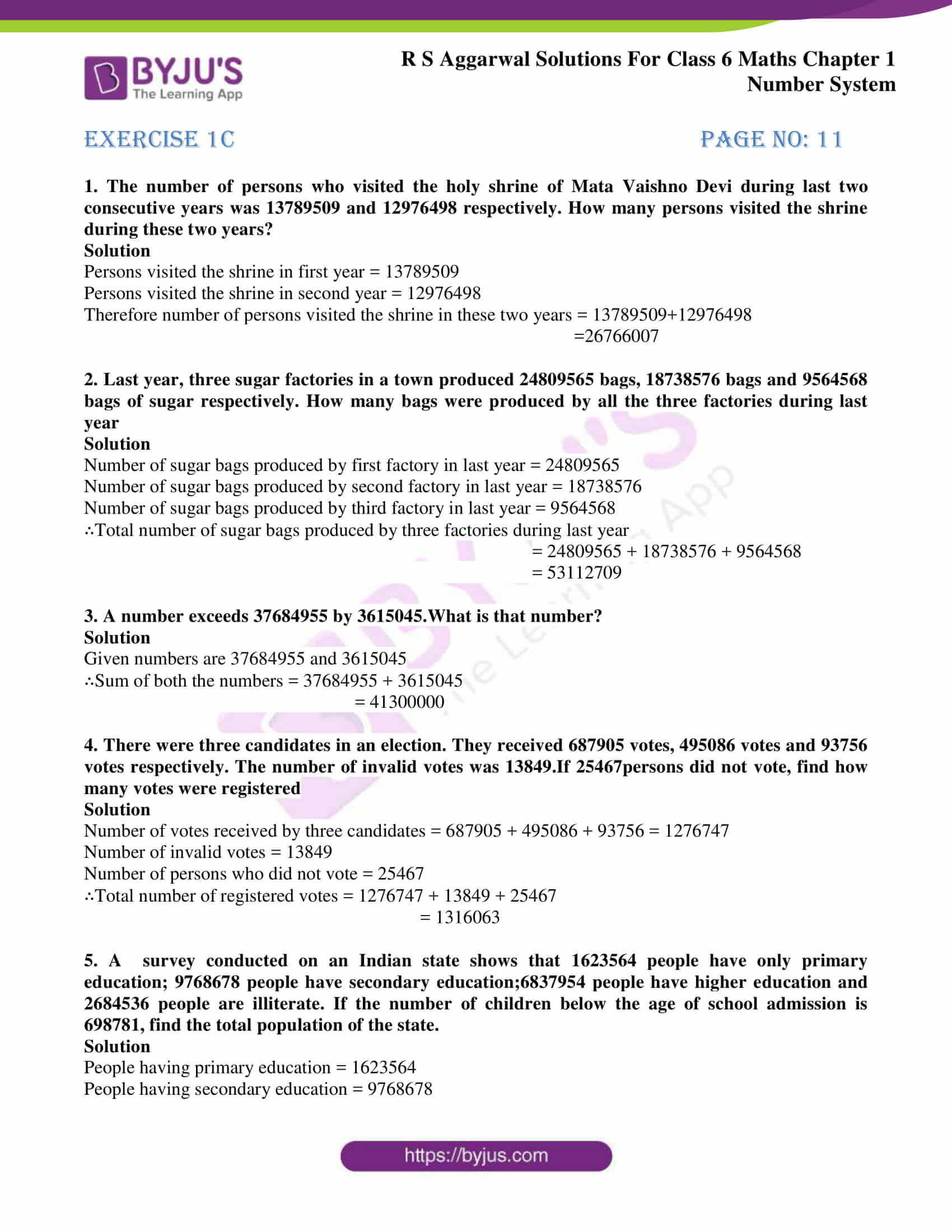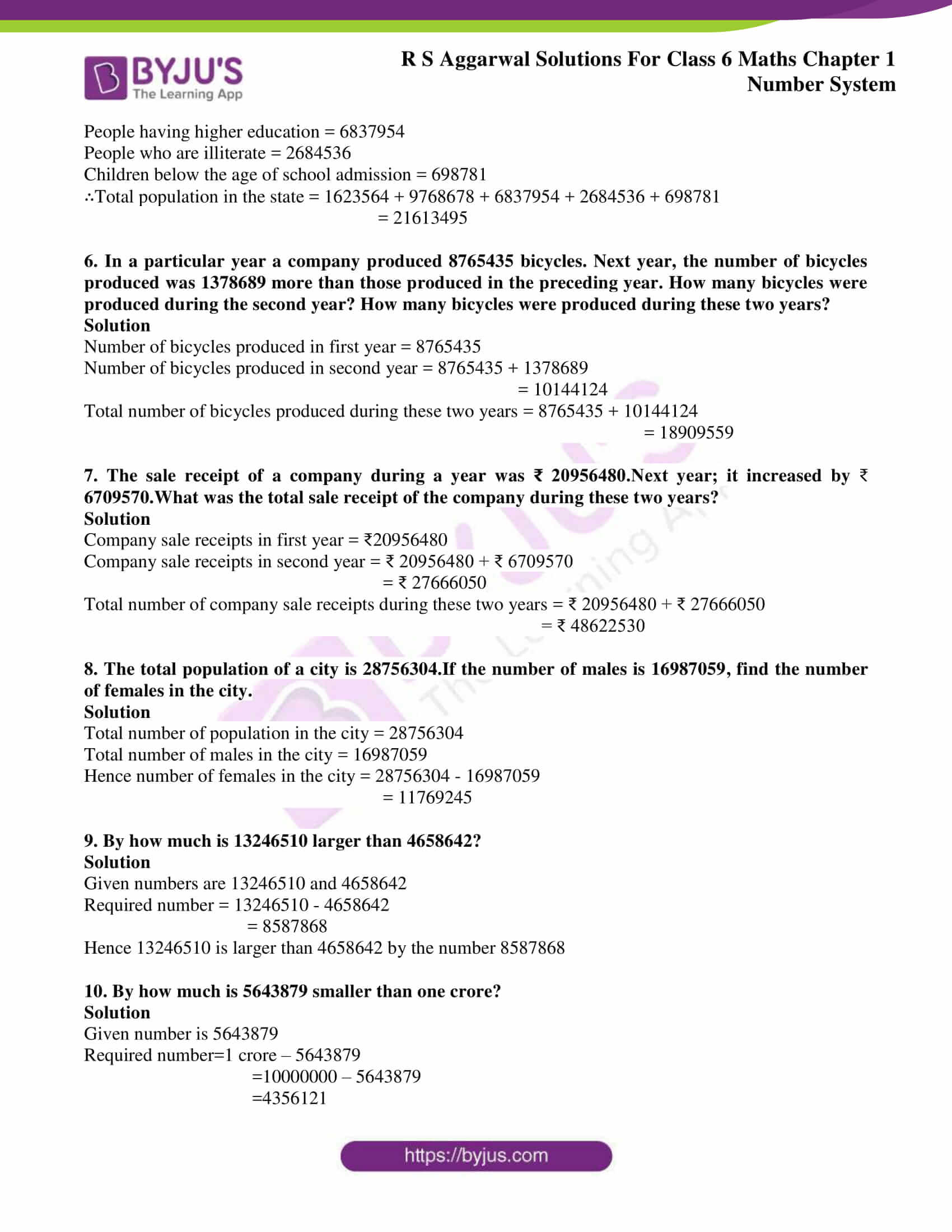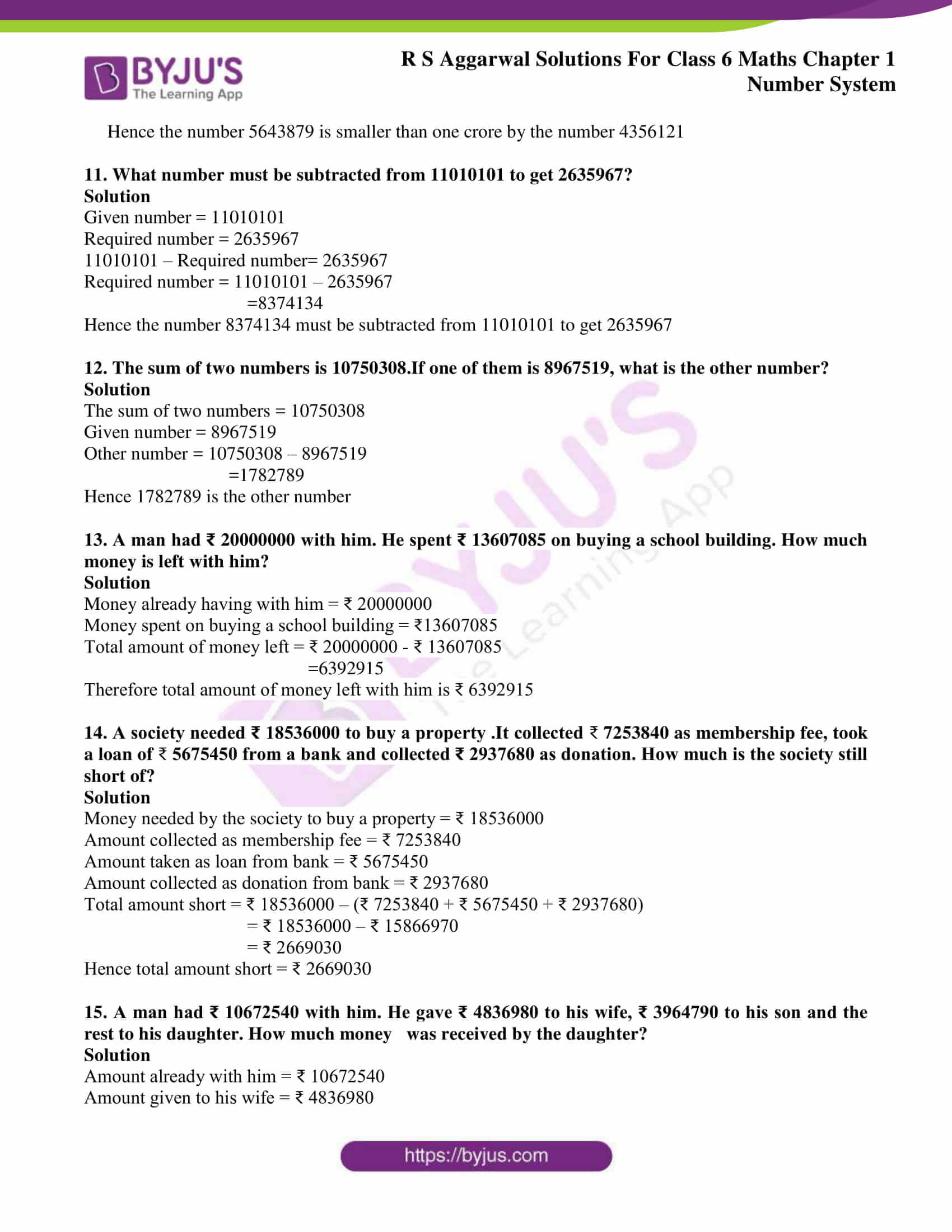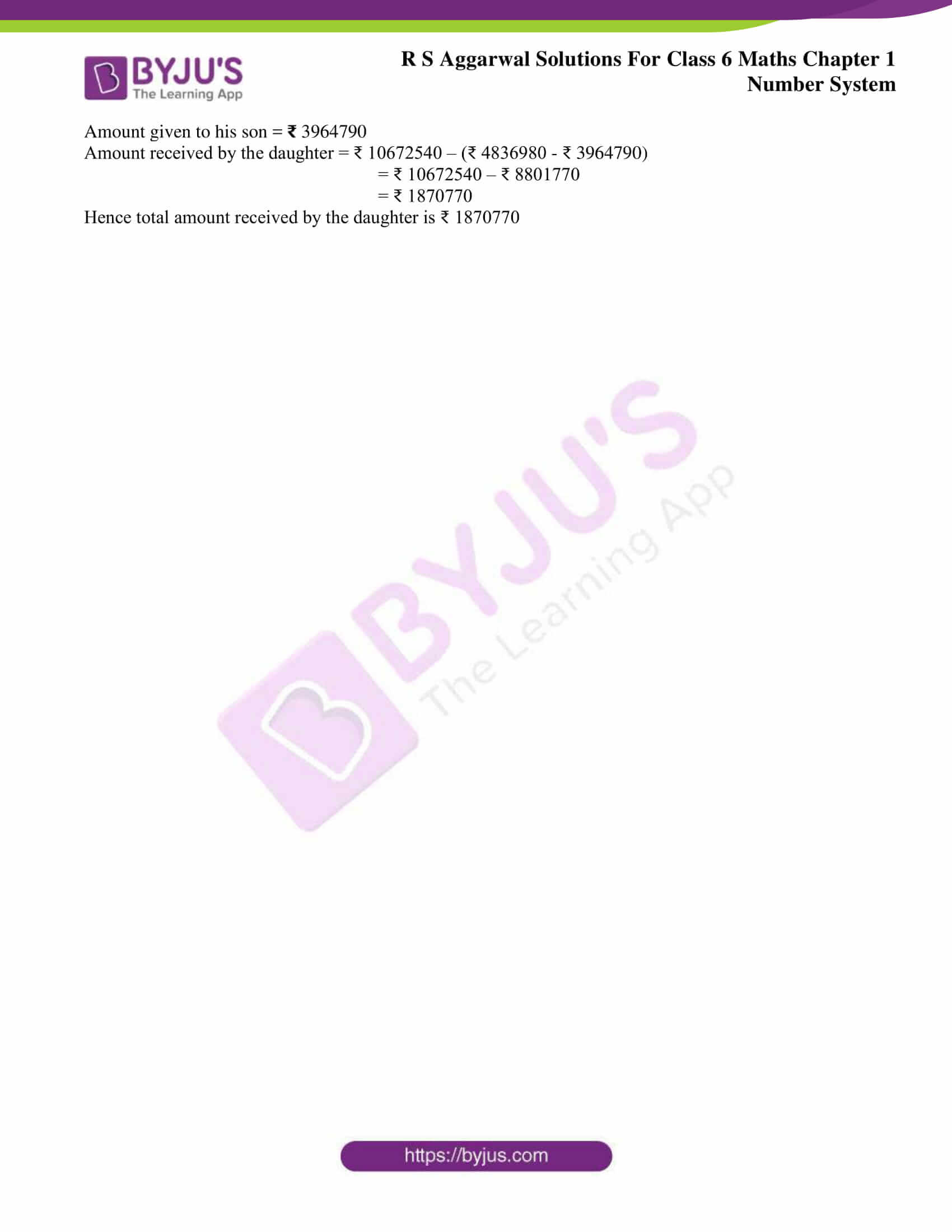# RS Aggarwal Solutions for Class 6 Chapter 1 Number System Exercise 1C

RS Aggarwal Solutions for Class 6, Chapter 1, Number System Exercise 1C has various word problems on number operations which are explained here. Experts in Mathematics have solved problems which are mentioned in Exercise 1C to help students solve problems with ease.

By practising RS Aggarwal Solutions for Class 6 Chapter 1 Number System, students easily understand the concepts explained under this chapter thoroughly. It mainly boosts their confidence in solving tricky problems and score high marks. Thus, students who aspire to improve their performance in the academic year are advised to go through RS Aggarwal Solutions for Class 6.

## Download PDF of RS Aggarwal Solutions for Class 6 Chapter 1 Number System Exercise 1C### Access answers to Maths RS Aggarwal Solutions for Class 6 Chapter 1 Number System Exercise 1C

1. The number of persons who visited the holy shrine of Mata Vaishno Devi during last two consecutive years was 13789509 and 12976498 respectively. How many persons visited the shrine during these two years?

Solution

Persons visited the shrine in first year = 13789509

Persons visited the shrine in second year = 12976498

Therefore number of persons visited the shrine in these two years = 13789509+12976498

=26766007

2. Last year, three sugar factories in a town produced 24809565 bags, 18738576 bags and 9564568 bags of sugar respectively. How many bags were produced by all the three factories during last year

Solution

Number of sugar bags produced by first factory in last year = 24809565

Number of sugar bags produced by second factory in last year = 18738576

Number of sugar bags produced by third factory in last year = 9564568

∴Total number of sugar bags produced by three factories during last year

= 24809565 + 18738576 + 9564568

= 53112709

3. A number exceeds 37684955 by 3615045.What is that number?

Solution

Given numbers are 37684955 and 3615045

∴Sum of both the numbers = 37684955 + 3615045

= 41300000

4. There were three candidates in an election. They received 687905 votes, 495086 votes and 93756 votes respectively. The number of invalid votes was 13849.If 25467persons did not vote, find how many votes were registered

Solution

Number of votes received by three candidates = 687905 + 495086 + 93756 = 1276747

Number of invalid votes = 13849

Number of persons who did not vote = 25467

∴Total number of registered votes = 1276747 + 13849 + 25467

= 1316063

5. A survey conducted on an Indian state shows that 1623564 people have only primary education; 9768678 people have secondary education;6837954 people have higher education and 2684536 people are illiterate. If the number of children below the age of school admission is 698781, find the total population of the state.

Solution

People having primary education = 1623564

People having secondary education = 9768678

People having higher education = 6837954

People who are illiterate = 2684536

Children below the age of school admission = 698781

∴Total population in the state = 1623564 + 9768678 + 6837954 + 2684536 + 698781

= 21613495

6. In a particular year a company produced 8765435 bicycles. Next year, the number of bicycles produced was 1378689 more than those produced in the preceding year. How many bicycles were produced during the second year? How many bicycles were produced during these two years?

Solution

Number of bicycles produced in first year = 8765435

Number of bicycles produced in second year = 8765435 + 1378689

= 10144124

Total number of bicycles produced during these two years = 8765435 + 10144124

= 18909559

7. The sale receipt of a company during a year was ₹ 20956480.Next year; it increased by 6709570.What was the total sale receipt of the company during these two years?

Solution

Company sale receipts in first year = ₹20956480

Company sale receipts in second year = ₹ 20956480 + ₹ 6709570

= ₹ 27666050

Total number of company sale receipts during these two years = ₹ 20956480 + ₹ 27666050

= ₹ 48622530

8. The total population of a city is 28756304.If the number of males is 16987059, find the number of females in the city.

Solution

Total number of population in the city = 28756304

Total number of males in the city = 16987059

Hence number of females in the city = 28756304 – 16987059

= 11769245

9. By how much is 13246510 larger than 4658642?

Solution

Given numbers are 13246510 and 4658642

Required number = 13246510 – 4658642

= 8587868

Hence 13246510 is larger than 4658642 by the number 8587868

10. By how much is 5643879 smaller than one crore?

Solution

Given number is 5643879

Required number=1 crore – 5643879

=10000000 – 5643879

=4356121

Hence the number 5643879 is smaller than one crore by the number 4356121

11. What number must be subtracted from 11010101 to get 2635967?

Solution

Given number = 11010101

Required number = 2635967

11010101 – Required number= 2635967

Required number = 11010101 – 2635967

=8374134

Hence the number 8374134 must be subtracted from 11010101 to get 2635967

12. The sum of two numbers is 10750308.If one of them is 8967519, what is the other number?

Solution

The sum of two numbers = 10750308

Given number = 8967519

Other number = 10750308 – 8967519

=1782789

Hence 1782789 is the other number

13. A man had ₹ 20000000 with him. He spent ₹ 13607085 on buying a school building. How much money is left with him?

Solution

Money already having with him = ₹ 20000000

Money spent on buying a school building = ₹13607085

Total amount of money left = ₹ 20000000 – ₹ 13607085

=6392915

Therefore total amount of money left with him is ₹ 6392915

14. A society needed ₹ 18536000 to buy a property .It collected 7253840 as membership fee, took a loan of 5675450 from a bank and collected ₹ 2937680 as donation. How much is the society still short of?

Solution

Money needed by the society to buy a property = ₹ 18536000

Amount collected as membership fee = ₹ 7253840

Amount taken as loan from bank = ₹ 5675450

Amount collected as donation from bank = ₹ 2937680

Total amount short = ₹ 18536000 – (₹ 7253840 + ₹ 5675450 + ₹ 2937680)

= ₹ 18536000 – ₹ 15866970

= ₹ 2669030

Hence total amount short = ₹ 2669030

15. A man had ₹ 10672540 with him. He gave ₹ 4836980 to his wife, ₹ 3964790 to his son and the rest to his daughter. How much money was received by the daughter?

Solution

Amount already with him = ₹ 10672540

Amount given to his wife = ₹ 4836980

Amount given to his son = 3964790

Amount received by the daughter = ₹ 10672540 – (₹ 4836980 – ₹ 3964790)

= ₹ 10672540 – ₹ 8801770

= ₹ 1870770

Hence total amount received by the daughter is ₹ 1870770

## RS Aggarwal Solutions for Class 6 Maths Chapter 1 Number System Exercise 1C

Exercise 1C of RS Aggarwal Solutions of Chapter 1 Number System consists of word problems on number operations. The solutions consists of step wise explanations in simple language which helps students in their exam preparation. Some of the topics which are explained in other exercises are given below:

• International system of numeration
• Comparison of numbers
• Estimation
• Estimating the products
• Estimating the quotients
• Roman numerals

Students are advised to follow RS Aggarwal Solutions to understand the Chapter in a better way as they provide solutions in a step by step manner. By practicing these solutions students will come out with good performance in the examinations